# Class 12 Maths NCERT Solutions for Chapter 5 Continuity and Differentiability Exercise 5.2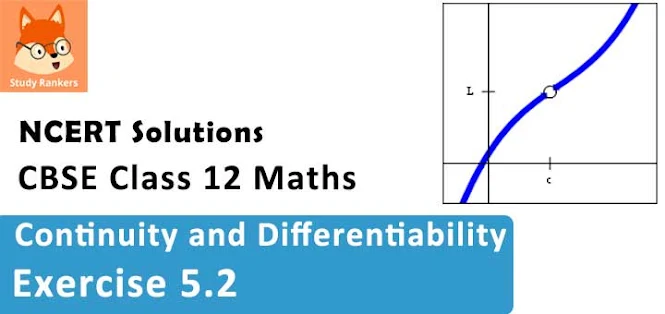### Continuity and Differentiability Exercise 5.2 Solutions

1. Differentiate the functions with respect to x.
sin (x2 + 5)

Solution

Let f(x) = sin (x2 + 5), u(x) = x2 + 5, and v(t) = sint
Then, (v o  u) (x) = v(u (x)) = v(x2 + 5) = sin(x2 + 5) = f(x)
Thus, f is a composite of two functions.
Put t = u(x) = x2 + 5
Then, we obtain
dv/dt = d/dt (sin t) = cost  = cos (x2 + 5)
dt/dx = d/dx (x2 + 5) = d/dx (x2 ) + d/dx (5) = 2x + 0 = 2x
Therefore by chain rule,
df/dx = dv/dt . dt/dx = cos (x2 + 5) × 2x =2x cos(x2 + 5)
Alternate method:2. Differentiate the functions with respect to x.
cos (sin x)

Solution

Let f(x) = cos (sin x), u(x) = sin x, and v(t) = cos t
Then, (vou)(x) = v(u(x)) = v(sin x) = cos (sin x) = f(x)
Thus, f is a composite function of two functions.
Put t = u (x) = sinx
∴ dv/dt = d/dt [cos t] = -sin t = -sin (sin x)
dt/dx = d/dx (sin x) = cos x
By chain rule,
df/dx = dv/dt . dt/dx = -sin (sin x). cos x = -cos x sin(sin x)
Alternate method:
d/dx [cos (sin x)] = -sin(sin x). d/dx (sin x) = -sin(sin x).cos x = -cos x sin(sin x)

3. Differentiate the functions with respect t x.
sin (ax + b)

Solution

Let f(x) = sin (ax + b), u(x) = ax + b, and v(t) = sin t
Then, (vou)(x) = v (u (x)) = v (ax + b) = sin(ax + b) = f(x)
Thus, f is a composite function of two functions, u and v.
Put t = u(x) = ax + b
Therefore,
dv/dt = d/dt (sin t) = cos t = cos (ax + b)
dt/dx = d/dx (ax + b) = d/dx (ax) + d/dx (b) = a + 0 = a
Hence by chain rule, we obtain
df/dx = dv/dt . dt/dx = cos (ax + b) . a = a cos (ax + b)
Alternate method: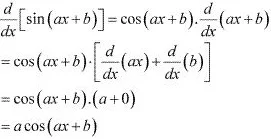4. Differentiate the function with respect to x.
sec(tan(√x))

Solution

Let f(x) = sec (tan√x), u(x) = √x, v(t) = tan t, and w(s) = sec s
Then, (wovou) (x) = w[v(u(x))] = w[v(√x)] = w(tan√x) = sec (tan√x) = f(x)
Thus, f is a composite function of three functions, u, v, and w.
Put s = v(t) = tan t and t = u(x) = √x
Then, dw/ds = d/ds (sec s) = sec s tan s  = sec (tan t). tan (tan t)  [s = tan t]
= sec (tan √x) . tan (tan √x)  [t = √x]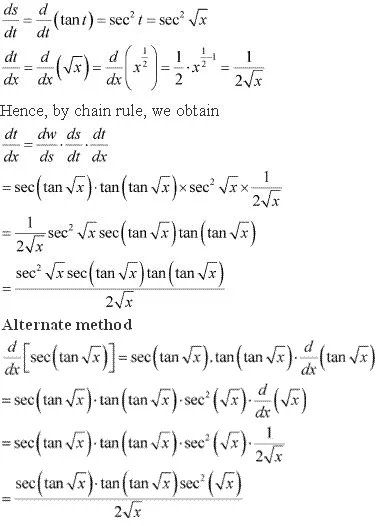5. Differentiate the functions with respect to x.
sin (ax + b)/cos (cx + d)

Solution

The given function is, where g(x) = sin (ax + b) and
h(x) = cos (cx + d)
∴ f ' = (g'h - gh')/h2
Consider g(x) = sin (ax + b)
Let u(x) = ax + b, v(t) = sin t
Then, (vou) (x) = v(u(x)) = v(ax + b) = sin (ax + b) = g(x)
∴ g is a composite function of two functions, u and v.
Put t = u(x) = ax + b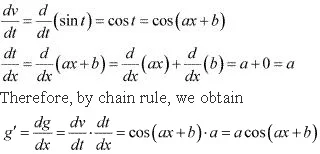Consider h(x) = cos (cx + d)
Let p(x) = cx + d, q(y) = cos y
Then, (qop)(x) = q (p(x)) = q(cx + d) = cos (cx + d) = h(x)
h is a composite function of two functions, p and q.
Put y = p(x) = cx +d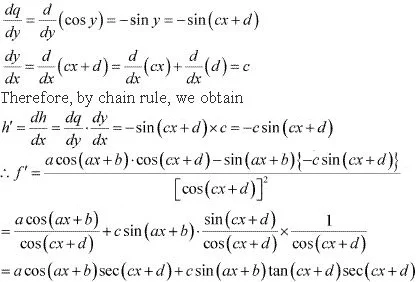6. Differentiate the functions with respect to x.
cos x3 . sin2 (x3 )

Solution

The given function is cos x3 . sin2 (x3)
d/dx [cos x3 .sin2 (x5)] = sin2 (x5) × d/dx( cos x3) + cos x3 × d/dx[sin2 (x5)]
= sin2 (x5) × (-sinx3) × d/dx(x3) + cos x3 × 2 sin(x5). d/dx [sinx5]
=  -sinx3 sin2 (x5) × 3x2 + 2 sinx5 cos x3 . cosx5 × d/dx(x5)
= - 3x2 sinx3 . sin2 (x5) + 2 sinx5 cos x5 cos x3 × 5x4
= 10 x4 sin x5 cos x5 cos x3 - 3x2 sin x3  sin2 (x5)

7. Differentiate the functions with respect to x.
2√cot(x2 )

Solution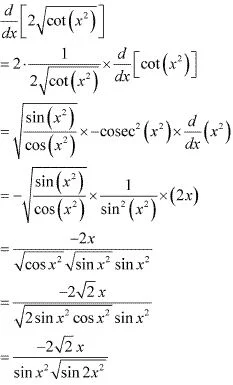8. Differentiate the functions with respect to x.
cos (√x)
Solution
Let f(x) = cos (√x)
Also, let u(x) = √x
And, v(t) = cos t
Then, (vou)(x) = v(u(x))
= v(√x)
= cos √x
= f(x)
Clearly, f is a composite function of two functions, u and v, such that
t = u(x) = √x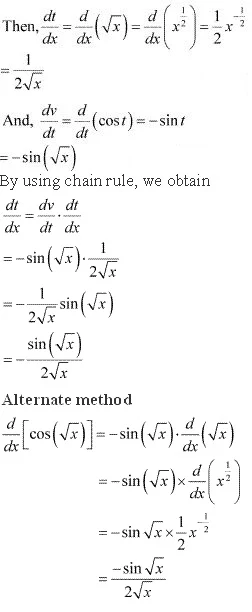9. Prove that the function f given by f(x) = |x - 1|, x ∊ R is not differentiable at x = 1.
Solution
The given function is f(x) = |x - 1|, x ∊ R
It is known that a function f is differentiable at a point x = c in its domain if both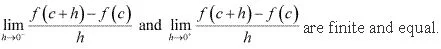To check the differentiability of the given function at x = 1,
consider the left hand limit of f at x = 1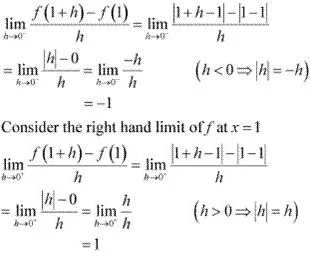Since the left and right  hand limits of f at x = 1 are not equal, f is not differentiable at x = 1

10. Prove that the greatest integer function defined by f(x) = [x], 0 < x < 3 is not differentiable at x = 1 and x = 2.
Solution
The given function f is f(x) = [x], 0 < x < 3
It is known that a function f is differentiable at a point x = c in its domain if both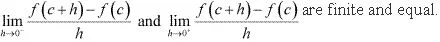To check the differentiability of the given function at x = 1, consider the left hand limit of f at x = 1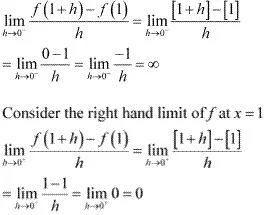Since  the left and right hand limits of f at x = 1 are not equal, f is not differentiable at x = 1
To check the differentiability of the given function at x = 2, consider the left hand limit of f at x = 2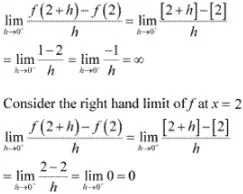Since the left and right hand limits of f at x = 2 are not equal, f is not differentiable at x = 2